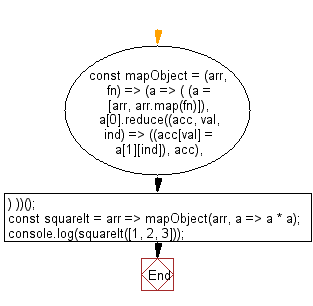# JavaScript: Map the values of an array to an object using a function

## JavaScript: Exercise-82 with Solution

Write a JavaScript program to map the values of an array to an object using a function, where the key-value pairs consist of the original value as the key and the mapped value.

Note: Use an anonymous inner function scope to declare an undefined memory space, using closures to store a return value. Use a new Array to store the array with a map of the function over its data set and a comma operator to return a second step, without needing to move from one context to another (due to closures and order of operations).

• Use Array.prototype.reduce() to apply fn to each element in arr and combine the results into an object.
• Use el as the key for each property and the result of fn as the value.

Sample Solution:

JavaScript Code:

``````//#Source https://bit.ly/2neWfJ2
const mapObject = (arr, fn) =>
(a => (
(a = [arr, arr.map(fn)]), a.reduce((acc, val, ind) => ((acc[val] = a[ind]), acc), {})
))();
const squareIt = arr => mapObject(arr, a => a * a);
console.log(squareIt([1, 2, 3]));
```
```

Sample Output:

```{"1":1,"2":4,"3":9}
```

Pictorial Presentation:Flowchart:Live Demo:

See the Pen javascript-basic-exercise-82-1 by w3resource (@w3resource) on CodePen.

Improve this sample solution and post your code through Disqus

What is the difficulty level of this exercise?

Test your Programming skills with w3resource's quiz.

﻿

## JavaScript: Tips of the Day

Chunks an array into n smaller arrays

Example:

```const tips_chunkIntoN = (arr, n) => {
const size = Math.ceil(arr.length / n);
return Array.from({ length: n }, (v, i) =>
arr.slice(i * size, i * size + size)
);
}
console.log(tips_chunkIntoN([1, 2, 3, 4, 5, 6, 7,8], 4));
```

Output:

```[[1,2],[3,4],[5,6],[7,8]]
```